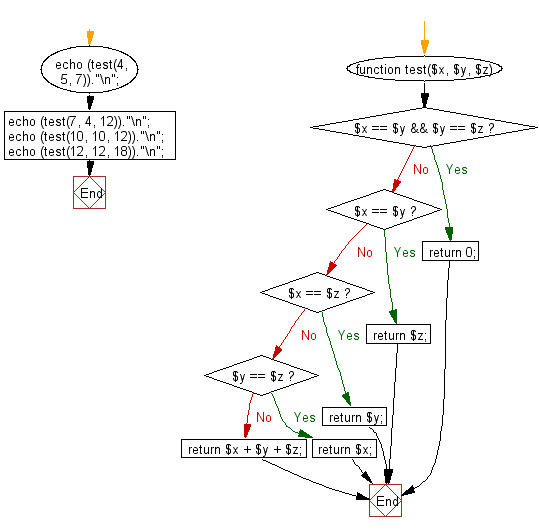﻿ PHP Exercises: Compute the sum of three given integers. If the two values are same return the third value - w3resource# PHP Exercises: Compute the sum of three given integers. If the two values are same return the third value

## PHP Basic Algorithm: Exercise-54 with Solution

Write a PHP program to compute the sum of three given integers. If the two values are same return the third value.

Sample Solution:

PHP Code :

``````<?php
function test(\$x, \$y, \$z)
{
if (\$x == \$y && \$y == \$z) return 0;
if (\$x == \$y) return \$z;
if (\$x == \$z) return \$y;
if (\$y == \$z) return \$x;
return \$x + \$y + \$z;
}
echo (test(4, 5, 7))."\n";
echo (test(7, 4, 12))."\n";
echo (test(10, 10, 12))."\n";
echo (test(12, 12, 18))."\n";
``````

Sample Output:

```16
23
12
18
```

Flowchart:PHP Code Editor:

What is the difficulty level of this exercise?

﻿

## PHP: Tips of the Day

\$GLOBALS: An associative array containing references to all variables which are currently defined in the global scope of the script. The variable names are the keys of the array

Example:

```<?php
\$myGlobal = "global"; // declare variable outside of scope
function test()
{
\$myLocal = "local"; // declare variable inside of scope
// both variables are printed
var_dump(\$myLocal);
var_dump(\$GLOBALS["myGlobal"]);
}
test(); // run function
// only \$myGlobal is printed since \$myLocal is not globally scoped

var_dump(\$myLocal);
var_dump(\$myGlobal);
?>
```

Output:

```string(5) "local"
string(6) "global"
NULL
string(6) "global"
```

In the above example \$myLocal is not displayed the second time because it is declared inside the test() function and then destroyed after the function is closed.

Becoming global

To remedy this there are two options.

Option one: global keyword

```function test()
{
global \$myLocal;
\$myLocal = "local";
var_dump(\$myLocal);
var_dump(\$GLOBALS["myGlobal"]);
}
```

The global keyword is a prefix on a variable that forces it to be part of the global scope.

Note that you cannot assign a value to a variable in the same statement as the global keyword. Hence, why I had to assign a value underneath. (It is possible if you remove new lines and spaces but I don't think it is neat. global \$myLocal; \$myLocal = "local").

Option two: \$GLOBALS array

```function test()
{
\$GLOBALS["myLocal"] = "local";
\$myLocal = \$GLOBALS["myLocal"];
var_dump(\$myLocal);
var_dump(\$GLOBALS["myGlobal"]);
}
```

In this example I reassigned \$myLocal the value of \$GLOBAL["myLocal"] since I find it easier writing a variable name rather than the associative array.